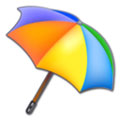Weather Word Search If you like a challenge then you’ll love our weather word search for kids. Do you have what it takes to find the range of words related to the wild world of weather? Try your hardest to see how many of the words you can find in this free puzzle game. This teaching resource is a fun and easy activity that will keep students happy and save teachers time.Printable Weather Word Search - Click this link for a printable version of the weather word search worksheet (opens in a new window). For the best printing results try adjusting the page setup in the print preview menu before printing. The 'Portrait' and 'Shrink to fit' settings usually work best.

Weather Word Search

Name _______________________ Date __________________

 H F Q O G C F E T L H E E A Y Z C E E S O O W R P H H Y C R S U N N Y N A S F R E L I A H K U Q F A S G A I T U C E E T O H K T V Y E P D S S F Z C Z C N C M V A T O R N A D O E F C E D I A E A R R M G Q J N C M A D M L A N O R E S P R E S S U R E S U R R O L I P S F T P L A F O P L O O O O A U M W U A E P K M Y A H W N L T G C E D G N L F H Q C O L D S Q C S R T X C Y W N C N Q F H J A N R O A E H K K K K U G E S L X M D O J B Q Y L K Z C T M D T N Y K E Y W C I

CLOUD
CLOUDY
COLD
FINE
FOG
FORECAST
FREEZE
HAIL
HEAT
HOT
PRESSURE
RAIN
SEASON
SNOW
STORM
SUN
SUNNY
TEMPERATURE